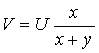SensorsIntroduction Architecture Hardware Results Our sensors Quantization

## Quantization ErrorMost of the sensors we are dealing with have a variable resistance (x). We are reading these by connecting a known bias resistor (y) in series. We can connect either x or y to the reference voltage, and the other end of the chain to the ground. The common point of the two resistances is read by the ADC. Assume that the ADC reading of the reference voltage is U, where U would be 1023 for a 10-bit converter and 4095 for a 12-bit one.

Let us assume that the unknown resistance (x) is at the bottom (see Figure 1). The ADC reading (V) will then beSolving for the value of x yieldsSince the ADC readings come in discrete steps, from 0 to U, let us consider how this quantization error affects our measurement. We compute the relative error in x, due to the quantization error, written as a symmetric expression in U and V. This can then be re-expressed as a function of r=(x/y). The error written this way will tell us how the bias resistor should be chosen to maximize the accuracy of the readings in a particular range of sensor resistance.It is clear, that the minimum of the error is at r=1, and the relative error changes in a symmetric fashion. The figure below shows how the relative quantization error behaves for U=1023 (10-bits) and U=4095 (12-bits).For a 10kOhm bias resistor, and a 10-bit converter we can get a 1% accurate measurement for sensor resistances in the 1.2 kOhm-80 kOhm range. For a 12 bit ADC we can cover the 250 Ohm- 400 kOhm range.